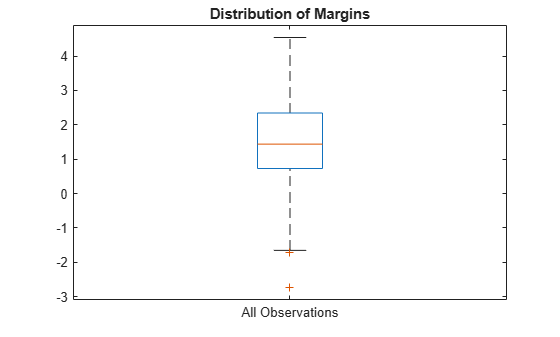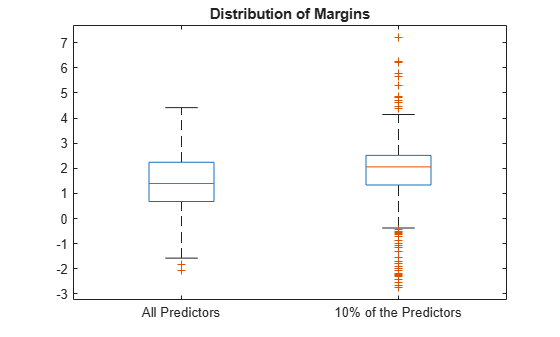kfoldMargin

Classification margins for cross-validated kernel classification model

Description

example

margin = kfoldMargin(CVMdl) returns the classification margins obtained by the cross-validated, binary kernel model (ClassificationPartitionedKernel) CVMdl. For every fold, kfoldMargin computes the classification margins for validation-fold observations using a model trained on training-fold observations.

Examples

collapse all

Load the ionosphere data set. This data set has 34 predictors and 351 binary responses for radar returns, which are labeled as either bad ('b') or good ('g').

Cross-validate a binary kernel classification model using the data.

CVMdl = fitckernel(X,Y,'Crossval','on')
CVMdl =
ClassificationPartitionedKernel
CrossValidatedModel: 'Kernel'
ResponseName: 'Y'
NumObservations: 351
KFold: 10
Partition: [1x1 cvpartition]
ClassNames: {'b'  'g'}
ScoreTransform: 'none'

Properties, Methods

CVMdl is a ClassificationPartitionedKernel model. By default, the software implements 10-fold cross-validation. To specify a different number of folds, use the 'KFold' name-value pair argument instead of 'Crossval'.

Estimate the classification margins for validation-fold observations.

m = kfoldMargin(CVMdl);
size(m)
ans = 1×2

351     1

m is a 351-by-1 vector. m(j) is the classification margin for observation j.

Plot the k-fold margins using a boxplot.

boxplot(m,'Labels','All Observations')
title('Distribution of Margins')Perform feature selection by comparing k-fold margins from multiple models. Based solely on this criterion, the classifier with the greatest margins is the best classifier.

Load the ionosphere data set. This data set has 34 predictors and 351 binary responses for radar returns, which are labeled either bad ('b') or good ('g').

Randomly choose 10% of the predictor variables.

rng(1); % For reproducibility
p = size(X,2); % Number of predictors
idxPart = randsample(p,ceil(0.1*p));

Cross-validate two binary kernel classification models: one that uses all of the predictors, and one that uses 10% of the predictors.

CVMdl = fitckernel(X,Y,'CrossVal','on');
PCVMdl = fitckernel(X(:,idxPart),Y,'CrossVal','on');

CVMdl and PCVMdl are ClassificationPartitionedKernel models. By default, the software implements 10-fold cross-validation. To specify a different number of folds, use the 'KFold' name-value pair argument instead of 'Crossval'.

Estimate the k-fold margins for each classifier.

fullMargins = kfoldMargin(CVMdl);
partMargins = kfoldMargin(PCVMdl);

Plot the distribution of the margin sets using box plots.

boxplot([fullMargins partMargins], ...
'Labels',{'All Predictors','10% of the Predictors'});
title('Distribution of Margins')The quartiles of the PCVMdl margin distribution are situated higher than the quartiles of the CVMdl margin distribution, indicating that the PCVMdl model is the better classifier.

Input Arguments

collapse all

Cross-validated, binary kernel classification model, specified as a ClassificationPartitionedKernel model object. You can create a ClassificationPartitionedKernel model by using fitckernel and specifying any one of the cross-validation name-value pair arguments.

To obtain estimates, kfoldMargin applies the same data used to cross-validate the kernel classification model (X and Y).

Output Arguments

collapse all

Classification margins, returned as a numeric vector. margin is an n-by-1 vector, where each row is the margin of the corresponding observation and n is the number of observations (size(CVMdl.Y,1)).

collapse all

Classification Margin

The classification margin for binary classification is, for each observation, the difference between the classification score for the true class and the classification score for the false class.

The software defines the classification margin for binary classification as

$m=2yf\left(x\right).$

x is an observation. If the true label of x is the positive class, then y is 1, and –1 otherwise. f(x) is the positive-class classification score for the observation x. The classification margin is commonly defined as m = yf(x).

If the margins are on the same scale, then they serve as a classification confidence measure. Among multiple classifiers, those that yield greater margins are better.

Classification Score

For kernel classification models, the raw classification score for classifying the observation x, a row vector, into the positive class is defined by

$f\left(x\right)=T\left(x\right)\beta +b.$

• $T\left(·\right)$ is a transformation of an observation for feature expansion.

• β is the estimated column vector of coefficients.

• b is the estimated scalar bias.

The raw classification score for classifying x into the negative class is f(x). The software classifies observations into the class that yields a positive score.

If the kernel classification model consists of logistic regression learners, then the software applies the 'logit' score transformation to the raw classification scores (see ScoreTransform).